# AP Physics C: Mechanics : Using Spring Equations

## Example Questions

2 Next →

### Example Question #42 : Mechanics Exam

A mass on the end of a spring oscillates back and forth. The period of an oscillating spring is measured to be 2.27 seconds. The mass is measured to be 0.300 kg. What is the spring's spring constant?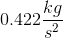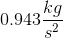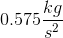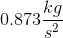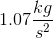Explanation:

To solve this problem, we can use the following equation: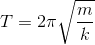Here,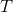is the period of an oscillation,is the mass of the oscillating thing in kg, andis the spring constant in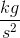.

Rearranging the equation to solve for the spring constant,, we get: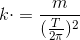Plugging in the two known values, we get: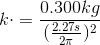Solving for, we get.

### Example Question #43 : Mechanics Exam

A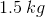block on a frictionless table is connected to a horizontal spring with constant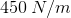. If the block is released from rest when the spring is stretched a distance of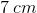,

what is its speed when the spring is compressed a distance of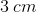?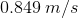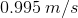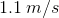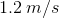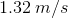Explanation:

Assuming this is a frictionless table, we don't have to take the work done by friction into account.

This is a conservation of energy problem. In this problem we have to realize that the potential energy of the spring atis equal to the kinetic energy of the spring at+ the potential energy of the spring at.

This can be shown in the following equation: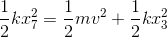If we solve for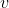, we get the following equation: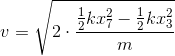Remember to convert the distances given in centimeters to meters.

If we plug in all the variables, we get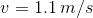2 Next →